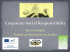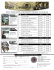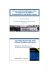Here - The Open University

Transcription

Here - The Open University
MATTER
Multivariate Analysis Today: Topical Expository Reviews
Programme and Book of abstracts
Scientific organisers: Frank Critchley (OU), Bing Li (Penn State), Hannu Oja (Turku)
Local organisers: Sara Griffin, Tracy Johns, Radka Sabolova, Germain Van Bever
Contents
Programme
3
Talk abstracts
5
John Kent: The Big Picture . . . . . . . . . . . . . . . . . . . . . . . . . . . . . . . . . . . . .
5
Siegfried H¨ormann: Functional Data Analysis . . . . . . . . . . . . . . . . . . . . . . . . . . .
6
David Hand: From Big Data to Beyond Data: Extracting the Value . . . . . . . . . . . . . .
8
Rajen Shah: Sparsity . . . . . . . . . . . . . . . . . . . . . . . . . . . . . . . . . . . . . . . . .
10
Hannu Oja: Non-parametric and Semi-parametric Methods . . . . . . . . . . . . . . . . . . .
12
Bing Li: Dimension Reduction . . . . . . . . . . . . . . . . . . . . . . . . . . . . . . . . . . .
14
Poster abstracts
17
On A Multivariate EWMA Control Chart Based on Spatial Sign Statistics . . . . . . . . . . .
17
Comparison of statistical methods for multivariate outliers detection . . . . . . . . . . . . . .
18
Flexible dimension reduction in regression . . . . . . . . . . . . . . . . . . . . . . . . . . . . .
19
Clustering multivariate data using central rank regions . . . . . . . . . . . . . . . . . . . . . .
20
On point estimation of the abnormality of a Mahalanobis distance . . . . . . . . . . . . . . .
21
Sparse Linear Discriminant Analysis with Common Principal Components . . . . . . . . . . .
22
Football and the dark side of cluster . . . . . . . . . . . . . . . . . . . . . . . . . . . . . . . .
23
Recovering Fisher linear discriminant subspace by Invariate Coordinate Selection . . . . . . .
24
Hilbertian Fourth Order Blind Identification . . . . . . . . . . . . . . . . . . . . . . . . . . . .
25
2
Programme
9:30
Registration and Coffee (Berrill Building)
10:20
Opening Remarks: Uwe Grimm (Head of OU Maths and Stats Dept)
10:30
John Kent (Leeds): The Big Picture
11:30
Siegfried H¨ormann (ULB): Functional Data Analysis
12:10
David Hand (Imperial College): Big Data
12:50
Pop-up Poster Presentations
13:00
Buffet Lunch and Poster Session
14:30
Rajen Shah (Cambridge): Sparsity
15:10
Hannu Oja (Turku): Non-parametric and Semi-parametric Methods
15:50
Bing Li (Penn State): Dimension Reduction
16:30
Tea and Departures (Berrill Building)
3
Talk abstracts
The Big Picture
John Kent, Department of Statistics, University of Leeds
Multivariate Analysis was originally developed as a largely “equivariant” set of procedures. That is,
any statistical inferences should be unchanged if the data undergo a nonsingular linear transformation.
Examples include Hotelling’s T 2 test, Fisher’s discriminant analysis and canonical variate analysis.
Also, many robustness methods (especially Tyler’s robust covariance matrix estimate), together with
methods such as invariant coordinate selection (ICS) and Independent Component Analysis (ICA) are
equivariant.
However, this appealing property comes at a price, especially when dealing with high dimensional
data. More modern methods relax the requirement for equivariance in order to increase the power
of the statistical methodology. There are several techniques of “regularization” through which “prior
beliefs” can be incorporated into the statistical analysis.
Some of the key ideas within regularization are: (a) a preferred metric, (b) a preferred choice of
variables, (c) shrinkage and (d) sparsity.
We shall show how these ideas underlie a variety of statistical tools ranging from the classic techniques of principal component analysis and factor analysis to the early modern method of ridge regression, and then to more modern methods such as LASSO, support vector machines, functional data
analysis based on splines and wavelets, and shape analysis.
5
Functional Data Analysis
Siegfried H¨ormann, Department of Mathematics, Universit´e libre de Bruxelles (ULB)
Data in many fields of science are sampled from processes that can most naturally be described
as functional. Examples include growth curves, temperature curves, curves of financial transaction
data and patterns of pollution data. Functional data analysis (FDA) is concerned with the statistical
analysis of such data. The purpose of this talk is to introduce some selected topics and to illustrate
them with a toy data set containing particulate matter concentrations.
Discrete to functional data
A functional observation X is of the form (X(t) : t ∈ T ) where T is some continuum. Mostly T is a
time interval (in which case we can and will assume that T = [0, 1]) and X(t) is then the value of some
random process at time t. More general functional domains, e.g. the surface of a sphere, are also not
uncommon but will not be considered here.
The first thing when it comes to analyze a functional sample (Xk : 1 ≤ k ≤ n) is to realize that
raw data are in general not functional. Rather we only sample from continuous-time data generating
processes (Xk (t) : t ∈ [0, 1]). The data can be sparsely observed or at high frequency, at regular time
points or irregularly, and they can come with or without measurement error. Hence, in a preliminary
step data need to be transformed in some meaningful way into curves. The most commonly used method
is the basis function approach in which a linear combination of some basis curves b1 (t), · · · , bq (t) is used
to approximate the discrete data. Often this can be simply achieved by a least squares approach, but
sometimes additional constraints, such as monotonicity, positivity or a certain degree of smoothness
may necessitate more sophisticated procedures. A further important issue is related to the fact that
physical time scale is not necessarily relevant for dynamics of a real-life system. Registration methods
can be used to deforming or shifting time such that curves become aligned.
Once the coefficients (Cki : 1 ≤ i ≤ q) in the representation
Xk (t) ≈
q
X
Cki bi (t)
i=1
are determined, we can use them to practically process the data with common multivariate tools. Ready
to use statistical software packages with many implemented features exist. Most notably the package
fda in R. An excellent introduction to practical aspects in FDA can be found in the text books of
Ramsay and Silverman  and .
Dimension reduction
Since observations are functions, we are dealing (at least in theory) with high-dimensional – in fact intrinsically infinite-dimensional – objects. So, not surprisingly, there is a demand for efficient dimensionreduction techniques. As such, functional principal component analysis (FPCA) has taken a leading
role in FDA, and functional principal components (FPC) arguably can be seen as the key technique in
the field.
6
In analogy to classical multivariate PCA, functional PCA relies on an eigendecomposition of the
underlying covariance function. Functional data can then be transformed into p-dimensional random
vectors by projecting onto the space spanned by eigenfunctions belonging to the p largest eigenvalues.
For smooth data it is then often possible to explain most of the variability by a few, say 3 or 4,
principal components. While with some background knowledge in functional analysis the procedure is
quite straight forward if data are fully observed, things can become involved if we have sparse data (see
e.g. Yao et al. ). It should be noted that an advantage of FPCA over its multivariate counterpart is
that FPCs do not suffer from the lack of scale invariance. Roughly speaking, while in the vector case
different components can have completely different measuring units, all points X(t), t ∈ [0, 1], of some
curve are expressed in the same units, and rescaling at different values of t is usually not meaningful.
Functional principal components are particularly useful for empirical data analysis, but they are also
heavily employed in statistical inference for functional data, including, among many others, FPC-based
estimation of functional linear models (e.g. Hall and Horowotiz ) or forecasting (e.g. Aue et al. ).
Functional regression
The functional linear model is given by the equation Y = Ψ(X) + ε where Ψ is a bounded linear
operator, X a functional observation and ε some error term which is independent of X. The response
Y is typically assumed to be either functional or scalar. In recent years this model has been intensively
studied in the literature from different perspectives. From an inferential point of view, the foremost
problem is to estimate the ‘regression operator’ Ψ. The two most popular methods of estimation are
based on principal component analysis (e.g., Hall and Horowitz ) and spline smoothing estimators
(e.g., Crambes et al. ). The crucial difficulty is that the infinite dimensional operator Ψ needs to be
ˆ K of finite dimension K, say. Clearly, K = Kn will depend on
approximated by a sample version Ψ
the sample size and tend to ∞ in order to obtain an asymptotically unbiased estimator. Tuning the
dimension parameter Kn is related to a bias-variance trade-off, but heavily relies on the (unknown)
spectrum of covariance operator of X. We will conclude this talk by discussing recent approaches which
allow to circumvent artificial assumptions commonly imposed in this context.
Bibliography
 Aue, A., Dubart Norinho, D. and H¨
ormann, S. (2015), On the prediction of stationary functional
time series, J. Amer. Statist. Assoc. 110, 378–392.
 Crambes, C., Kneip, A. and Sarda, P. (2009), Smoothing splines estimators for functional linear
regression, The Annals of Statistics, 37, 35–72.
 Hall, P. and Horowitz, J. (2007), Methodology and convergence rates for functional linear regression,
The Annals of Statistics, 35, 70–91.
 Ramsay, J. and Silverman, B. (2002), Applied Functional Data Analysis, Springer, New York.
 Ramsay, J. and Silverman, B. (2005), Functional Data Analysis (2nd ed.), Springer, New York.
 Reiss, P. T. and Ogden, R. T. (2007), Functional principal component regression and functional
partial least squares, J. Amer. Statist. Assoc. 102, 984–996.
 Yao, F., M¨
uller, H.G., and Wang, J.-L. (2005), Functional linear regression analysis for longitudinal
data, The Annals of Statistics, 33, 2873–2903.
7
From Big Data to Beyond Data: Extracting the Value
David J. Hand, Imperial College, London
The phrase “big data has taken the media by storm in recent years, with dramatic promises for
scientific breakthroughs, economic growth, and medical advances. As a McKinsey report put it “we
are on the cusp of a tremendous wave of innovation, productivity, and growth, as well as new modes of
competition and value capture–all driven by big data as consumers, companies, and economic sectors
exploit its potential” (Manyika et al., 2011), or, as Stephan Shakespeare said “from data we will get
the cure for cancer as well as better hospitals; schools that adapt to childrens needs making them
happier and smarter; better policing and safer homes; and of course jobs. Data allows us to adapt and
improve public services and businesses and enhance our whole way of life, bringing economic growth,
wideranging social benefits and improvements in how government works ... the new world of data is
good for government, good for business, and above all good for citizens” (Shakespeare, 2013).
But are these grandiose claims justified? Is the world really going to be transformed? Or, as is
the case with any advanced technology (think nuclear or biological), do advances come with technical
challenges, ethical conundrums, and conflicting aims? Bracketing all of this is the observation that
no-one wants data: what people want are answers. Data is not information, and information is not
knowledge, so big data, per se, is not sufficient.
Big data is typically defined along various continua: number of cases, number of dimensions, number
of modes, complexity, and so on. One implication of this is that relatively small data sets, in terms of
mere number of observations, might be big in other terms: genomic problems based on a few hundred
individuals might be big in terms of the tens of thousands of gene expression observations and the
power set of potential interactions between them.
Many conceptually different kinds of challenges have been lumped together under the neologism
“big data”. I find it useful to divide these into two types: data manipulation and inference. These two
types provide opportunities for different kinds of breakthroughs.
Data manipulation is concerned with sorting, adding, searching, matching, concatenating, aggregating, and so on. Examples of recent innovations based on such tools include automatic route finders,
apps for bus and trains status, and tools for identifying a piece of music. The challenges are mathematical and computing, often involving how to reduce the time for an operation from millions of years
to something practicable, or reduce the required storage to something feasible.
Inference is concerned with using the available data to make a statement about some other or
some more extensive population. In medical research, for example, our aim is typically not to draw
conclusions about the patients we have in front of us, but about all patients with the condition. In
economic forecasting, we use past data to try to make a statement about the future.
The phrase n = all has been used to describe the potential of big data. This often relates to
administrative data, data collected automatically during the course of some activity: tax records, credit
card purchases, education scores, licence details, etc. But the phrase is misleading it has been described
as “the big misconception” (McIvor, 2014). The fundamental underlying point is that no data set is
perfect. Indeed, the problems of data quality and missing data have been extensively investigated with
“small” data sets, and they are inevitably worse with large data sets.
8
Inferential analysis of “big data” depends critically on a sound understanding of statistical concepts
and methods. Without this, dramatically mistaken conclusions can be drawn, as I illustrate with a
series of real examples, each of which has attracted considerable media coverage.
Apart from the practical challenges, there are also ethical uncertainties. These arise most obviously
in the realms of data security, privacy, and confidentiality. It is very apparent that the legal system is
struggling to keep up: I shall say a little about UK and EU attempts to draw up suitable compromises
in the context of data sharing.
Bibliography
 Manyika J., Chui M., Brown B., Bughin J., Dobbs R., Roxburgh C., and Byers R.H.
(2011), Big data:
the next frontier for innovation, competition, and productivity.
http://www.mckinsey.com/insights/business technology/big data the next frontier for innovation
 McIvor N. (2014) Personal communication
 Shakespeare S. (2103) Shakespeare Review: An independent review of public sector information,
https://www.gov.uk/government/publications/shakespeare-review-of-public-sector-information
9
Sparsity
Rajen D. Shah, Statistical Laboratory, University of Cambridge
Classical statistics was designed for datasets with a large (but not overwhelming) number of observations of a few carefully chosen variables. In recent years, however, as statisticians we’ve been
challenged with an increasing number of datasets of very different shape and size, for which we have
needed to develop, and indeed still need to invent, radically different new tools to perform data analysis.
Sparsity has been at the centre of much of this statistical research. The word is used to mean different
ideas in different contexts, but perhaps the two most important forms of sparsity are what one might
term signal sparsity and data sparsity.
Signal sparsity
Consider a regression context with n observations (Yi , xi ) ∈ R × Rp , i = 1, . . . , n with Yi the response
and xi a p-dimensional covariate vector. Signal sparsity refers to an assumption that most of the p
predictors are unrelated to the response. Specialising to a linear model for the data,
Yi = µ + xTi β 0 + εi ,
this translates to the coefficient vector β 0 being sparse i.e. most of its components are 0.
Though such sparse models are unlikely to be exactly true, they are nevertheless useful for a great
number of modern datasets, particularly in the so-called high-dimensional setting where the number of
predictors p greatly exceeds the number of observations n. Microarray datasets, where the number of
variables (gene expression values) may number in the tens of thousands, and the number of observations
(tissue samples) could be a few hundred at best, are a prototypical example.
When p > n (so X does not have full column rank), the estimate of β 0 from ordinary least squares
(OLS) will not be unique and it will overfit to the extent that the fitted values will equal those observed.
The famous Lasso estimator  addresses this problem by adding an `1 penalty term to the least squares
ˆ and estimated intercept µ
objective criterion, with the estimated regression coefficients, β,
ˆ, satisfying
ˆ = arg min { 1 kY − µ1 − Xβk2 + λkβk1 },
(ˆ
µ, β)
2
2n
(1)
µ,β
P
where 1 denotes an n-vector of 1’s and kβk1 := pk=1 |βk |. The tuning parameter, λ > 0, trades off the
complexity of the fitted model with the fidelity of the fit to Y . Due to the form of the penalty on β,
a large number of components of βˆ will be exactly 0. This sparsity property of the estimator helps it
deliver sensible estimates even when p n.
An enormous amount of research has been directed at extending the Lasso in various ways, and
studying its theoretical properties. See B¨
uhlmann and van de Geer  for some of these developments,
and references therein. In terms of theory, asymptotic arguments where n → ∞ whilst p remains fixed
cannot usually be relied upon to be relevant in finite samples where p n. Instead finite sample
results are often obtained. An important result concerning the Lasso establishes that with λ chosen
10
appropriately and independent errors εi ∼ N (0, σ 2 ), one has with high probability
r
log(p)
0
kβˆ − β k1 ≤ constant × s σ
n
under conditions on the design matrix that in particular disallow certain variables from being too correlated with one another. More recently, inferential procedures based on the Lasso have been produced
which, for example, can give confidence intervals for coefficients βk0 .
Data sparsity
Many modern datasets fall within the high-dimensional “large p, small n” setting. However, primarily
from industry, we are also seeing datasets where both the numbers of observations and predictors
can number in the millions or (much) more. Where in high-dimensional data the main challenge is
a statistical one was more parameters must be estimated than there are observations available, in
this “big data” setting there are also serious computational issues. Here OLS may be infeasible for
computational, rather than statistical, reasons.
An important feature of many of these large-scale datasets, such as those arising from a bag-ofwords representation of a corpus of documents, is that the overwhelming majority of entries in the
design matrices are exactly zero: the data matrices are sparse. Successful methods in this setting
exploit this sparsity for computational and statistical gains.
Given the scale of the data, a sensible way to proceed is by first performing dimension reduction, that
is mapping the original design matrix X ∈ Rn×p to S ∈ Rn×L with L p. This dimension reduction
step must be computationally fast and ideally should scale linearly with the number of non-zeroes in
the design matrix. Developing methods that perform well under this computational constraint is a
rather young area of statistical research. However, the computer science literature does have a variety
of what are known sometimes known as sketching or hashing algorithms for performing this step.
One of the most prominent approaches is to form S via random projections of X. The simplest
version of this technique effectively forms S through S = XA where the entries in A are i.i.d. standard
normals. Another interesting approach that is particularly suited to the sparse setting and is applicable
when the design matrix is binary is b-bit min-wise hashing , which is based on an earlier technique
called min-wise hashing . This technique is studied from a statistical perspective in Shah and
Meinshausen , where an extension to continuous data is also introduced.
Bibliography
 Broder, A., Charikar, M., Frieze, A. and Mitzenmacher, A. (1998) Min-wise independent permutations. Proceedings of the thirtieth annual ACM symposium on theory of computing, 327–336.
 B¨
uhlmann, P. and van de Geer, S. (2011) Statistics for High-Dimensional Data: Methods, Theory
and Algorithms. Springer, Springer Series in Statistics.
 Li, P. and K¨
onig, A.C. (2011) Theory and applications of b-bit min-wise hashing. Communications
of the ACM, 54, 101–109.
 Shah, R.D. and Meinshausen, N. (2015) Min-wise hashing for large-scale regression and classification
with sparse data. arXiv preprint.
 Tibshirani, R. (1996) Regression shrinkage and selection via the Lasso J. Roy. Statist. Soc., Ser.
B, 58, 267–288.
11
Non-parametric and Semi-parametric Methods
Hannu Oja, University of Turku
In this talk we review some non-parametric and semi-parametric methods to analyze continuous
multivariate data. First, natural semi-parametric models, the so called location-scatter models, are described that include the multivariate normal model, the elliptic model and the independent component
model as special cases. Then different concepts of multivariate signs and ranks and the statistical properties of the induced tests and estimates are discussed with a special focus on the model assumptions.
The talk ends with a short discussion on semi-parametric dimension reduction methods.
Multivariate Semi-parametric Models
Semi-parametric location-scatter models for an observed p-vector x are obtained if one assumes that
x = µ + Ωz,
where µ is the location vector, Ω is the mixing matrix, and z is an unobservable standardized random
variable. One then assumes, for example, that E(z) = 0 and Cov(z) = Ip so that E(x) = µ and
Cov(x) = Σ = ΩΩT . One can further assume that
(i) z ∼ Np (0, Ip ) (multivariate normal model),
(ii) ||z|| ⊥
⊥ ||z||−1 z (elliptic model) or
(iii) z1 ⊥
⊥ z2 ⊥
⊥ ... ⊥
⊥ zp (independent component model)
Note that (ii) and (iii) provide to different semi-parametric extensions of the multivariate normal model.
Multivariate Signs and Ranks
The univariate concepts of sign and rank are based on the ordering of the data. Unfortunately, in the
multivariate case there are no natural orderings for the data points. An approach utilizing L1 criterion
functions is therefore often used to extend the concepts of sign and rank to the multivariate case. Let
x1 , ..., xn be a random sample from a multivariate location-scatter model. The multivariate signs ui
and multivariate (centered) ranks ri , i = 1, ..., n, are then implicitly defined using the L1 criterion
functions
n
n
n
n
n
1X
1X T
1 XX
1X T
||xi || =
ui xi and
||xi − xj || =
ri x i .
n
n
2n
n
i=1
i=1
i=1 j=1
i=1
Notice that the sign and centered rank may be seen as location scores Ti = T(xi ) and the model
parameters µ and Σ are defined to satisfy E(T(zi )) = 0 and Cov(T(zi )) = Ip with zi = Σ−1/2 (xi − µ).
(T(x) = x is the score function for the regular L2 criterion and gives the regular mean vector and
covariance matrix.)
The extensions of the concepts of sign and rank then depend on the chosen L1 norm. Manhattan
and Euclidean norms give the vectors of marginal signs and ranks and the so called spatial sign and
12
rank, correspondingly. In this talk, the statistical properties of different extensions are discussed in the
light of previous model assumptions. The affine equivariance/invariance of the procedures is obtained
by transforming the data to an invariant coordinate system (ICS). See Tyler et al. (2009), Oja (2010)
and Ilmonen et al. (2012).
Semi-parametric models and dimension reduction
In dimension reduction, one often asks whether there is a p × k matrix B or corresponding projection
matrix P = B(BT B)−1 BT , such that BT x carries all the relevant information and, depending on the
problem at hand, this has lead to more specific questions such as
(i) Px carries most of the variation of x (PCA),
(ii) Px and (Ip − P)x are the non-Gaussian and Gaussian parts of the variation (ICA), or
(iii) x ⊥
⊥ y|Px (SIR, SAVE, etc.) for some interesting response variable y.
We again discuss the roles of semi-parametric model assumptions and invariant coordinate selection in
Bibliography
 Ilmonen, P., Oja, H. and Serfling, R. (2012). On Invariant Coordinate System (ICS) Functionals.
International Statistical Review, 80, 93-110.
 Liski, E., Nordhausen, K. and Oja, H. (2014). Supervised Invariant Coordinate Selection. Statistics:
A Journal of Theoretical and Applied Statistics, 48, 711-731.
 Multivariate Nonparametric Methods with R. An Approach Based on Spatial Signs and Ranks.
Springer, New York
 Tyler, D., Critchley, F., Dumbgen, L., and Oja, H. (2009). Invariant coordinate selection. Journal
of Royal Statistical Society, B, 71, 549–592.
13
Dimension Reduction
Bing Li and Jun Song, The Pennsylvania State University
We propose a general theory and the estimation procedures for nonlinear sufficient dimension reduction where
the predictor or the response, or both, are random functions. The relation between the response and predictor
can be arbitrary and the sets of observed time points can vary from subject to subject. The functional and
nonlinear nature of the problem leads naturally to consideration of two layers of functional spaces: the first
space consisting of functions of time; the second space consisting of functions defined on the first space. We take
both spaces to be reproducing kernel Hilbert spaces. A particularly attractive feature of our construction is that
the two functional spaces are nested, so that the kernel for the first space determines the kernel for the second.
We propose two estimators, functional generalized sliced inverse regression (f-GSIR) and functional genearlized
sliced average variance estimator (f-GSAVE) for this general dimension reduction problem. We investigated the
performances of our estimators by simulations, and applied them to data sets about phoneme recognition and
handwritten symbols.
Sufficient dimension reduction
Sufficient dimension reduction (SDR) is characterized by conditional independence
Y
X|β T X,
(2)
where X is a p-dimensional random vector, Y is a random variable, and β is a p×d matrix (d p). The
goal is to estimate the space spanned by the columns of β. That is, we seek a few linear combinations
of X that are sufficient to describe the conditional distribution of Y given X [see 8, 3]. Sufficient
dimension reduction problem (2) is linear in the sense that the reduced predictor takes the linear form
β T X. For this reason, we will refer to it as linear sufficient dimension reduction. Linear SDR was
generalized to functional data by Ferr´e and Yao , and Hsing and Ren .
The theory of linear SDR was generalized to the nonlinear case by Li, Artemiou, and Li  and Lee,
Li, and Chiaromonte . Nonlinear SDR seeks a set of nonlinear functions f1 (X), . . . , fd (X) such that
Y
X|f1 (X), . . . , fd (X).
(3)
This was accomplished by enlarging the Euclidean space of linear coefficient vectors for linear SDR to
a Hilbert space of functions of X. Lee, Li, and Chiaromonte  showed that the nonlinear functions
f1 , . . . , fq in (3) can be obtained from the eigenfunctions of certain linear operators, and developed
two methods to estimate them. The precursors this general theory include Bach and Jordan , Cook
, Wu , and Yeh, Huang, and Lee , which introduced a variety of practical nonlinear sufficient
dimension reduction methods without articulating a unifying framework.
Sufficient dimension reduction for functional data
We go one step further to develop a theory and estimation methods for nonlinear sufficient dimension
reduction for functional data. Let X and Y be random functions defined on a set T in a finitedimensional Euclidean space. Assume X and Y reside in a Hilbert space H. Our goal is to find a set
of nonlinear functionals f1 , . . . , fd on H to R such that the random functions Y and X are independent
conditioning on the random variables f1 (X), . . . , fd (X). The functional and nonlinear nature of this
14
problem demands that we consider two nested functional spaces. First, X and Y are themselves
functions of T , and they reside in functional spaces whose domains are T . Second, f1 (X), . . . , fd (X)
reside in a space of functions whose domains are the first space.
To understand the delicate structures of these two layers of spaces with clarity, and to explore their
inter relation and come up with estimators of f1 , . . . , fd would be the core of this paper.
This generalization is motivated and justified by many recent applications. For example, consider the
problem of training the computer to learn to associate two sets of handwritten symbols, one numerical
and one alphabetical, as illustrated in Figure 1.
←→
←→
In this problem both the predictor and the response are in the form of two dimensional functions
t 7→ (f1 (t), f2 (t))T , which describe the curves in a two dimensional space. Moreover, the relation is too
complicated to be described through linear index as in classical sufficient dimension reduction. The
methods proposed here are sufficiently flexible to handle all these situations.
Bibliography
 Bach, F. R. and Jordan, M. I. (2002), “Kernel Independent Component Analysis,” Journal of
Machine Learning Research, 3, 1–48.
 Cook, R. D. (2007), “Fisher lecture: Dimension reduction in regression,” Statistical Science, 22,
1–26.
 Cook, R. D. and Weisberg, S. (1991), “Sliced Inverse Regression for Dimension Reduction: Comment,” 86, 328–332.
 Ferr´e, L. and Yao, A. F. (2003), “Functional sliced inverse regression analysis,” Statistics: A
Journal of Theoretical and Applied Statistics, 37, 475–488.
 Hsing, T. and Ren, H. (2009), “An RKHS formulation of the inverse regression dimension-reduction
problem,” The Annals of Statistics, 37, 726–755.
 Lee, K.-Y., Li, B., and Chiaromonte, F. (2013), “A general theory for nonlinear sufficient dimension
reduction: Formulation and estimation,” The Annals of Statistics, 41, 221–249.
 Li, B., Artemiou, A., and Li, L. (2011), “Principal support vector machines for linear and nonlinear
sufficient dimension reduction,” The Annals of Statistics, 39, 3182–3210.
 Li, K.-C. (1991), “Sliced inverse regression for dimension reduction,” Journal of the American
Statistical Association, 86, 316–327.
 Wu, H.-M. (2008), “Kernel Sliced Inverse Regression with Applications to Classification,” Journal
of Computational and Graphical Statistics, 17, 590–610.
 Yeh, Y.-R., Huang, S.-Y., and Lee, Y.-J. (2009), “Nonlinear Dimension Reduction with Kernel
Sliced Inverse Regression,” IEEE Transactions On Knowledge And Data Engineering, 11, 1590–
1603.
15
Poster abstracts
On A Multivariate EWMA Control Chart Based on Spatial Sign
Statistics
To maintain the quality of a product or to improve the reliability of a process, all industries need to
monitor several parameters about their production process. Control charts are some visualization tools
for monitoring processes statistically. They have been in use in the manufacturing processes for a quite
long time, but all of them were based on either a single characteristic of the process or they used several
different charts for different characteristics ignoring the dependence between the characteristics. With
the ease of computing power and advances in technology, it is now easier to monitor several characteristics at the same time and to include their interdependencies as well. In this project, we propose a few
control charting schemes to monitor several characteristics of a process at the same time and to detect
when it goes out of control. Our objective is to reduce the false alarms (the scheme detects a problem
when actually there is none) as well as to quickly detect the correct out-of-control situation. The novelty of the proposed schemes are that they do not depend on commonly assumed Normal distribution
of the process variables and is applicable for a much wider range of data distributions.
17
Comparison of statistical methods for multivariate outliers detection
Aurore Archimbaud1 , Klaus Nordhausen2 & Anne Ruiz-Gazen1
1
2
Gremaq (TSE), Universit´e Toulouse 1 Capitole,
E-mail: [email protected]
[email protected]
Department of Mathematics and Statistics, University of Turku,
E-mail: [email protected]
In this poster, we are interested in detecting outliers, like for example manufacturing defects, in
multivariate numerical data sets. Several non-supervised methods that are based on robust and nonrobust covariance matrix estimators exist in the statistical literature. Our first aim is to exhibit the
links between three outliers detection methods: the Invariant Coordinate Selection method as proposed
by Caussinus and Ruiz-Gazen (1993) and generalized by Tyler et al. (2009), the method based on
the Mahalanobis distance as detailed in Rousseeuw and Van Zomeren (1990), and the robust Principal
Component Analysis (PCA) method with its diagnostic plot as proposed by Hubert et al. (2005).
Caussinus and Ruiz-Gazen (1993) proposed a Generalized PCA which diagonalizes a scatter matrix
relative to another: V1 V2−1 where V2 is a more robust covariance estimator than V1 , the usual empirical
covariance estimator. These authors compute scores by projecting V2−1 -orthogonally all the observations
on some of the components and high scores are associated with potential outliers. We note that
computing euclidean distances between observations using all the components is equivalent to the
computation of robust Mahalanobis distances according to the matrix V2 using the initial data. Tyler
et al. (2009) generalized this method and called it Invariant Coordinate Selection (ICS). Contrary to
Caussinus and Ruiz-Gazen (1993), they diagonalize V1−1 V2 which leads to the same eigen elements but
to different scores that are proportional to each other. As explained in Tyler et al. (2009), the method is
equivalent to a robust PCA with a scatter matrix V2 after making the data spherical using V1 . However,
the euclidean distances between observations based on all the components of ICS corresponds now to
Mahalanobis distances according to V1 and not to V2 .
Note that each of the three methods leads to a score for each observation and high scores are associated
with potential outliers. We compare the three methods on some simulated and real data sets and
show in particular that the ICS method is the only method that permits a selection of the relevant
components for detecting outliers.
Keywords. Invariant Coordinate Selection; Mahalanobis distance; robust PCA.
Bibliography
 Caussinus, H. and Ruiz-Gazen, A. (1993), Projection pursuit and generalized principal component
analysis, In New Directions in Statistical Data Analysis and Robustness (eds S. Morgenthaler, E.
Ronchetti and W. A. Stahel), 35–46, Basel: Birkh¨auser.
 Hubert, M., Rousseeuw, P. J. and Vanden Branden, K. (2005), ROBPCA: a new approach to robust
principal component analysis, Technometrics, 47(1), 64–79.
 Rousseeuw, P. J. and Van Zomeren, B. C. (1990), Unmasking multivariate outliers and leverage
points, Journal of the American Statistical Association, 85(411), 633–639.
 Tyler, D. E., Critchley, F., D¨
umbgen, L. and Oja, H. (2009), Invariant coordinate selection, Journal
of the Royal Statistical Society: Series B (Statistical Methodology), 71(3), 549–592.
18
Flexible dimension reduction in regression
Andreas Artemiou, Cardiff University
Sufficient Dimension Reduction (SDR) is a class of methods that have been used for to reduce the
dimensions of a regression problem. If we have a p dimensional predictor vector X, the main objective
is to find d (where d is less than p) linear or nonlinear directions which if they are used as predictors
in the model there will be no loss of information on the conditional distribution of Y |X (where Y is
the response). This led to numerous algorithms being presented in the last 25 years. Many methods
have also used inverse moments to perform SDR in regression problems. Our focus will be on algorithms like Sliced Inverse Regression (SIR - Li 1991) and Cumulative Mean Estimation (CUME - Zhu
Zhu and Feng 2010) which use the first inverse moment E(X|Y ). The main drawback of SIR was
the necessity to tune for the number of slices, where the main drawback for CUME was it’s performance for regression modes with a categorical response. In this poster we will discuss new method
which can be implemented using two different algorithms. One is equivalent to SIR and the other is
equivalent to CUME and therefore it has the ability to be tuned based ans select one of the two algorithms to be performed. Also it provides different insights to the asymptotic theory of the two methods.
19
Clustering multivariate data using central rank regions
Mohammed Baragilly, Birmingham University
A problem with cluster analysis is to determine the optimal number of clusters in the multivariate
data. Over the last 40 years, a wealth of publications has been developed, introduced and discussed
many graphical approaches and statistical algorithms in order to determine the optimal number of
clusters. Here, we propose a novel method that can be considered as an exploratory tool for clustering
multivariate data and determining the suitable number of clusters. One of the common ways to determine the number of clusters is the detection of outliers in a sample of multivariate data. The forward
search algorithm is one of the graphical approaches that lead to the formal detection of multivariate
outliers and consequently determining the expected number of clusters. The traditional forward search
approach based on Mahalanobis distances has been introduced by Hadi (1992) and Atkinson (1994),
while Atkinson et al. (2004) used it as a clustering method. In many statistical analyses some nonparametric multivariate methods as spatial signs and ranks, and central rank regions are usually used to
describe and solve the multivariate data problems and to get techniques which are less sensitive to the
statistical model assumptions. More robust results can be obtained by using the ranks instead of the
original values. For instance, we can get more information for each observation about how central it is
and in which direction it is moving from the center, this is due to that length of Rank(x) tells us how
far away this point is from the center and the direction of Rank(x) tells us about the direction of x from
the centre of the data. Serfling (2002) proposed the concept of volume functional based on central rank
regions. He considered the spatial quantiles, introduced by Chaudhuri (1996) and Koltchinskii (1997)
as a certain form of generalization of the univariate case based on the L1 norm. We propose a new
forward search methodology based on spatial ranks, which provides a visualization tool of cluster sizes,
where clusters are grown with one data point at a time sequentially, using spatial ranks with respect to
the points already in the subsample. The algorithm starts from a randomly chosen initial subsample.
We illustrate that the proposed algorithm is robust to the choice of initial subsample and it outperforms
forward search based on Mahalanobis distances for mixture bivariate Laplace and t distributions under
spherical and elliptic symmetry. We also propose a forward search methodology based on the volume
of central rank regions. The results of our numerical examples show that it gives the best results under
both spherical and elliptic symmetry. Finally, we illustrate our methodology using a real data set with
econometric application.
20
On point estimation of the abnormality of a Mahalanobis distance
1
2
The Open University
University of Aberdeen
Email: [email protected]
When a patient appears to have unusual symptoms, measurements or test scores, the degree to
which this patient is unusual becomes of interest. For example, clinical neuropsychologists sometimes
need to assess how a patient with some brain disorder or a head injury is different from the general
population or some particular subpopulation. This is usually based on the patient’s scores in a set
of tests that measure different abilities. Then, the question is “What proportion of the population
would give a set of test scores as extreme as that of the patient?” The abnormality of the patient’s
profile of scores is expressed in terms of the Mahalanobis distance between his profile and the average
profile of the normative population. The degree to which the patient’s profile is unusual can then be
equated to the proportion of the population who would have a larger Mahalanobis distance than the
individual. This presentation will focus on forming an estimator of this proportion using a normative
sample. The estimators that are examined include plug-in maximum likelihood estimators, medians,
the posterior mean from a Bayesian probability matching prior, an estimator derived from a Taylor
expansion, and two forms of polynomial approximation, one based on Bernstein polynomial and one
on a quadrature method. Simulations show that some estimators, including the commonly-used plugin maximum likelihood estimators, can have substantial bias for small or moderate sample sizes. The
polynomial approximations yield estimators that have low bias, with the quadrature method marginally
to be preferred over Bernstein polynomials. Moreover, simulations of the median estimators have a
nearly zero median error. This latter estimator has much to recommend it when unbiasedness is not of
paramount importance, while the quadrature method is recommended when bias is the dominant issue.
Keywords. Bernstein polynomials; Mahalanobis distance; median estimator; quadrature approximation; unbiased estimation.
21
Sparse Linear Discriminant Analysis with Common Principal
Components
Tsegay G. Gebru & Nickolay T. Trendafilov
Department of Mathematics and Statistics, The Open University, UK
Linear discriminant analysis (LDA) is a commonly used method for classifying a new observation
into one of g-populations. However, in high-dimensional classification problems the classical LDA has
poor performance. When the number of variables is much larger than the number of observations,
the within-group covariance matrix is singular which leads to unstable results. In addition, the large
number of input variables needs considerable reduction which nowadays is addressed by producing
sparse discriminant functions.
Here, we propose a method to tackle the (low-sample) high-dimensional discrimination problem by
using common principal components (CPC). LDA based on CPC is a general approach to the problem
because it does not need the assumption of equal covariance matrix in each groups. We find sparse
CPCs by modifying the stepwise estimation method proposed by Trendafilov (2010). Our aim is to find
few important spare discriminant vectors which are easily interpretable. For numerical illustrations,
the method is applied on some known real data sets and compared to other methods for sparse LDA.
Bibliography
 Trendafilov, N.T. Stepwise estimation of common principal components. Computational Statistics
and Data Analysis 54:3446-3457, 2010.
22
Football and the dark side of cluster
Christian Hennig, University College London
In cluster analysis, decisions on data preprocessing such as how to select, transform, and standardise
variables and how to aggregate information from continuous, count and categorical variables cannot
be made in a supervised manner, i.e., based on prediction of a response variable. Statisticians often
attempt to make such decisions in an automated way by optimising certain objective functions of the
data anyway, but this usually ignores the fact that in cluster analysis these decisions determine the
meaning of the resulting clustering. We argue that the decisions should be made based on the aim
and intended interpretation of the clustering and the meaning of the variables. The rationale is that
preprocessing should be done in such a way that the resulting distances, as used by the clustering
method, match as well as possible the “interpretative distances” between objects as determined by the
meaning of the variables and objects. Such “interpretative distances” are usually not precisely specified
and involve a certain amount of subjectivity. We will use ongoing work on clustering football players
based on performance data to illustrate how such decisions can be made, how much of an impact they
can have, how the data can still help with them and to highlight some issues with the approach.
23
Recovering Fisher linear discriminant subspace by Invariate
Coordinate Selection
Radka Sabolov´a1,2 , H. Oja3 , G. Van Bever1 & F. Critchley1 .
1
MCT Faculty, The Open University, Milton Keynes
2 Email: [email protected]
3 Turku University
It is a remarkable fact that, using any pair of scatter matrices, invariant coordinate selection (ICS)
can recover the Fisher linear discriminant subspace without knowing group membership, see . The
subspace is found by using two different scatter matrices S1 and S2 and joint eigendecomposition of
one scatter matrix relative to another.
In this poster, we focus on the two group normal subpopulation problem and discuss the optimal
choice of such a pair of scatter matrices in terms of asymptotic accuracy of recovery. The first matrix
is fixed as the covariance matrix while the second one is chosen within a one-parameter family based
on powers of squared Mahalanobis distance, indexed by α ∈ R. Special cases of this approach include
Fourth Order Blind Identification (FOBI, see ) and Principal Axis Analysis (PAA, see ).
The use of two scatter matrices in discrimination was studied by  and later elaborated in , who
proposed generalised PCA (GPCA) based on a family of scatter matrices with decreasing weight functions of a single real parameter β > 0. They then discussed appropriate choice of β, while concentrating
on outlier detection.
Their form of weight function and the consequent restriction to β > 0 implies downweighting outliers.
On the other hand, in our approach, considering any α ∈ R allows us also to upweight outliers. Further,
we may, in addition to the outlier case, study mixtures of subpopulations.
Theoretical results are underpinned by an extensive numerical study.
The UK-based authors thank the EPSRC for their support under grant EP/L010429/1.
Bibliography
 Cardoso, J.-F. Source Separation Using Higher Moments Proceedings of IEEE international conference on acoustics, speech and signal processing 2109-2112.
 Caussinus, H. and Ruiz-Gazen, A. Projection pursuit and generalized principal component analyses
New direction in Statistical Data Analysis and Robustness 35-46.
 Caussinus, H., Fekri, M., Hakam, S. and Ruiz-Gazen, A. A monitoring display of multivariate
outliers Computational Statistics & Data Analysis, 2003, 44, 237–252.
 Critchley, F., Pires, A. and Amado, C. Principal Axis Analysis technical report, Open University,
2006.
 Tyler, D., Critchley, F., Dumbgen, L. and Oja, H. Invariant Co-ordinate Selection J. R. Statist.
Soc. B., 2009, 71, 549–592.
24
Hilbertian Fourth Order Blind Identification
Germain Van Bever1,2 , B. Li3 , H. Oja4 , R. Sabolov´a1 & F. Critchley1 .
1
MCT Faculty, The Open University, Milton Keynes
2 Email: [email protected]
3 Penn State University
4 Turku University
In the classical Independent Component (IC) model, the observations X1 , · · · , Xn are assumed to
satisfy Xi = ΩZi , i = 1, . . . , n, where the Zi ’s are i.i.d. random vectors with independent marginals
and Ω is the mixing matrix. Independent component analysis (ICA) encompasses the set of all methods
aiming at unmixing X = (X1 , . . . , Xn ), that is estimating a (non unique) unmixing matrix Γ such that
ΓXi , i = 1, . . . , n, has independent components. Cardoso () introduced the celebrated Fourth Order
Blind Identification (FOBI) procedure, in which an estimate of Γ is provided, based on the regular
covariance matrix and a scatter matrix based on fourth moments. Building on robustness considerations and generalizing FOBI, Invariant Coordinate Selection (ICS, ) was originally introduced as an
exploratory tool generating an affine invariant coordinate system. The obtained coordinates, however,
are proved to be independent in most IC models.
Nowadays, functional data (FD) are occurring more and more often in practice, and relatively
few statistical techniques have been developed to analyze this type of data (see, for example ).
Functional PCA is one such technique which focuses on dimension reduction with very little theoretical
considerations. We propose an extension of the FOBI methodology to the case of Hilbertian data,
FD being the go-to example used throughout. When dealing with distributions on Hilbert spaces, two
major problems arise: (i) the scatter operator is, in general, non-invertible and (ii) there may not exist
two different affine equivariant scatter functionals. Projections on finite dimensional subspaces and
Karhunen-Lo`eve expansions are used to overcome these issues and provide an alternative to FPCA.
More importantly, we show that the proposed construction is Fisher consistent for the independent
components of an appropriate Hilbertian IC model and enjoy the affine invariance property.
This work is supported by the EPSRC grant EP/L010429/1.
Keywords. Invariant Coordinate Selection; Functional Data; Symmetric Component Analysis;
Independent Component Analysis.
Bibliography
 Cardoso, J.-F. (1989), Source Separation Using Higher Moments Proceedings of IEEE international
conference on acoustics, speech and signal processing 2109-2112.
 Tyler, D., Critchley, F., Dumbgen, L. and Oja, H. (2009) Invariant Co-ordinate Selection J. R.
Statist. Soc. B., 71, 549–592.
 Ramsay, J. and Silverman, B.W. (2006) Functional Data Analysis 2nd edn. Springer, New York.
25

Math Analysis Honors – Worksheet 44 Multivariate Linear Systems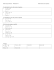Analyzing Multivariate Count Data Using a GQL Based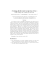Workshop On Multivariate Analysis Today Programme and Book of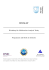CHIMIOMÃTRIE 2016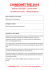Assignment 2B-2: Regression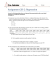NESS2015sc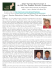A new technology that has you protected Simple and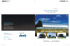Jan Dvořáček Technical University in Zvolen